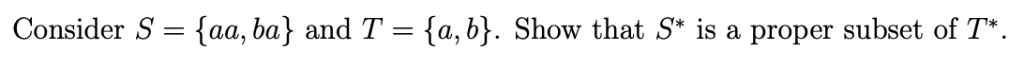# If A is a subset of B and B is a subset of C, can you conclude that A is a subset of C

If A is a subset of B and B is a subset of C, can you conclude that A is a subset of C? Can you conclude that A is a proper subset of C?
If A is a proper subset of B and B is a proper subset of C, can you conclude that A is a subset of C? Can you conclude that A is a proper subset of C?
If A is a subset of B and B is a proper subset of C, can you conclude that A is a subset of C? Can you conclude that A is a proper subset of C?

This Homework Help Question: "If A is a subset of B and B is a subset of C, can you conclude that A is a subset of C" No answers yet.

We need 3 more requests to produce the answer to this homework help question. Share with your friends to get the answer faster!

0 /3 have requested the answer to this homework help question.

Once 3 people have made a request, the answer to this question will be available in 1-2 days.
All students who have requested the answer will be notified once they are available.
##### Add Answer of: If A is a subset of B and B is a subset of C, can you conclude that A is a subset of C
Similar Homework Help Questions
• ### If A is a subset of B (not necessarily proper) and B is a subset of C (not necessarily proper) the A is a proper subset of C

If A is a subset of B (not necessarily proper) and B is a subset of C (not necessarily proper) the A is a proper subset of C.True or Falseif false must provide a counterexample

• ### If A is a subset of B (not necessarily proper) and B is a subset of C (not necessarily proper) the A is a proper subset of C

If A is a subset of B (not necessarily proper) and B is a subset of C (not necessarily proper) the A is a proper subset of C. True or Falseif false must provide a counterexample

• ### Suppose B is proper subset of C

Suppose B is proper subset of C.If n(c)=8, what is the maximum number of elements n B?What is the least possible number of elements B?

• ### If A is a finite set and B is a proper subset of A, prove that |B| < |A|

If A is a finite set and B is a proper subset of A, prove that |B| < |A|. Hint: B is finite. What is the union of the disjoint sets B and A - B? Note: |A| = size of A

• ### Need help with this subset problem Consider S aa, ba and T- {a, b}. Show that S is a proper subset of T. Consider S aa, ba and T- {a, b}. Show that S is a proper subset of T.Need help with this subset problem Consider S aa, ba and T- {a, b}. Show that S is a proper subset of T. Consider S aa, ba and T- {a, b}. Show that S is a proper subset of T.

• ### Part 1 I have to use symbols in my anwser Suppose B is proper subset of C If n(c)=8, what is the maxium number of elements in n (B) What is the least possible numbers of Elements in B

Part 1 I have to use symbols in my anwser Suppose B is proper subset of CIf n(c)=8, what is the maxium number of elements in n (B)What is the least possible numbers of Elements in B?Part 2Suppose C is a subset of D and D is a subset of CIf n (C)=5, find n (D) What is other relationship exists between sets C and D?

• ### Suppose C is a subset of D and D is a Subset of C

Suppose C is a subset of D and D is a Subset of C.If n(c)=5,find n(D)what other relationship exists between C and D

• ### suppose c is a subset of d and d is a subset of c

suppose c is a subset of d and d is a subset of c. if n(c) = 5, find n (d).

• ### I have to make a true statement with this question: {All states west of the Rocky Mountains} {All states west of the Atlantic Ocean} I have to determine if the two statements are: Proper Subset is a subset of is not a subset of proper subset and is

I have to make a true statement with this question:{All states west of the Rocky Mountains} {All states west of theAtlantic Ocean}I have to determine if the two statements are: Proper Subsetis a subset ofis not a subset ofproper subset and is a subset of

• ### Let f:A->B, where A and B are nonempty, and let T1 and T2 be subsets of B

Let f:A->B, where A and B are nonempty, and let T1 and T2 be subsets of B.a.Prove that f^-1(T1 U T2)= f^-1(T1) U f^-1(T2).b.Prove that f^-1(T1 intersects T2) = f^-1(T1) intersects f^-1(T2). I think once I see a I can do b.c. Prove that f^-1(T1) - f^-1(T2) = f^-1(T1-T2).d. Prove that if T1 is a proper subset of T2, then f^-1(T1) is a proper subset of f^-1(T2).I am confused on this one and need some help understanding inverse mapping. Thank...

Need Online Homework Help?